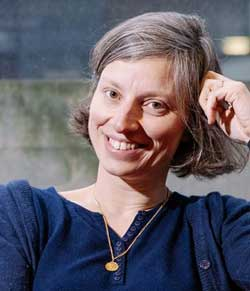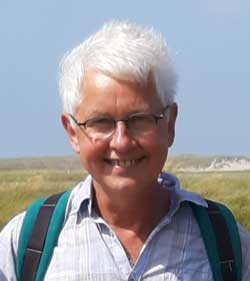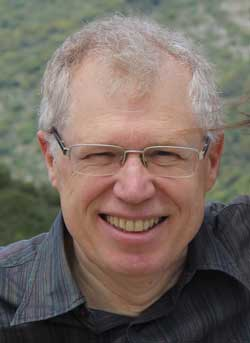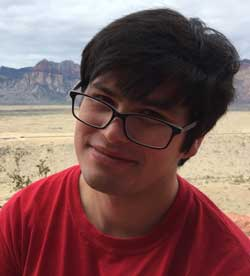# Laure Saint Raymond

## Laure Saint Raymond | École Normale Supérieure de Lyon

Over the past three decades, geophysical flows have aroused a lot of interest in the mathematical community. The specificity of these flows is due to the combination of rotation and stratification. Their phenomenology is therefore very rich, first of all because there is a large range of parameters (viscosity, compressibility, salinity, density,…) and also because geometry plays an important role.

The mathematical study of these multi-scale problems appeals to various techniques : filtering of waves, compensated compactness, boundary layers, homogenization, pseudo-differential calculus (with strongly nonlocal effects), … We will present here some results in the case of weakly viscous incompressible rotating fluids.

1. Taylor Proudman columns : "rotation stabilizes the fluid around the 2D flow…
2. Ekman pumping : "rotation stabilizes the boundary layer in the inviscid limit…
3. Attractors : "when inertial waves meet topography…

## Susan Friedlander | University of Southern California

#### The Joy of Small Parameters

Many equations that model fluid behavior are derived from systems that encompass multiple physical forces. When the equations are written in non dimensional form appropriate to the physics of the situation, the resulting PDEs often involve multiple non-dimensional parameters. Frequently some of these parameters are very small and they enter into the analysis in different ways. We will discuss one such system which has been proposed as a model for magnetostrophic turbulence and describe results that can be obtained in several different small parameter limits. In this talk we will concentrate on a forced drift-diffusion equation for the temperature where the fluid viscosity enters via the drift velocity. We examine the convergence of solutions in the limit as the viscosity goes to zero. We introduce a natural notion of ”vanishing viscosity ” weak solutions and prove the existence of a compact global attractor for the critical drift-diffusion equation.

This is joint work with Anthony Suen.

## Pierre Germain | Courant (NYU)

#### vortex filament solutions of Navier-Stokes

I will present a recent result obtained in collaboration with J. Bedrossian and B. Harrop-Griffiths. We prove that the Navier-Stokes equations, in 3 dimensions, are locally well-posed if the vorticity of the initial data is supported on a smooth curve. Besides its physical relevance, this result is also the first one to explore the local well-posedness of Navier-Stokes in a neighborhood of large self-similar data.

## Leo R.M. Maas | Institute for Marine and Atmospheric research Utrecht, Utrecht University, the Netherlands

#### Inertial waves: the constraints of confinement

A homogeneous-density fluid that is in solid-body rotation is characterized by a stable stratification in angular momentum. Perturbations manifest themselves as inertial waves, characterized by a dispersion relation that relates wave frequency to wave vector inclination only. This is typical for anisotropic waves. It implies inertial waves behave in a manner complementary to familiar surface gravity waves. This is evident especially when the boundaries of the fluid domain are also inclined relative to the direction of the rotation axis. In this case inertial waves approach limit cycles, called wave attractors. In two-dimensional settings, inertial waves, while linear, display spatial, spectral and parametric self-similarity – features usually held synonymous with nonlinear dynamical systems. They can be characterized by a topological invariant, and are recognized as a broad-band phenomenon. The extension of geometric and analytical two-dimensional solution methods to three-dimensions is cumbersome. In three dimensions, laboratory experiments show the presence of an attracting two-dimensional manifold. Inertial wave ray patterns suggest that, upon a further symmetry-breaking of its container’s shape, this may collapse on a one-dimensional “super-attractor”. Due to wave focusing at attractors, angular momentum is mixed, driving a mean flow parallel to iso-angular momentum surfaces.

## Alex Mahalov | Arizona State University

#### Stochastic Three-Dimensional Navier-Stokes Equations + Waves: Resonances, Averaging, Convergence, Regularity and Nonlinear Dynamics

We consider nonlinear interactions described by the stochastic three-dimensional Navier- Stokes equations coupled with waves dynamics. Regularity results are established by bootstrapping from global regularity of the averaged stochastic resonant equations and convergence theorems. The averaged covariance operator couples stochastic and wave effects. The energy injected in the system by the noise is large, the initial condition has large energy, and the regularization time horizon is long. Infinite time regularity is proven for the deterministic case. Regularization is the consequence of precise mechanisms of relevant three-dimensional nonlinear interactions. In the periodic case, these 3D nonlinear interactions are described using algebraic geometry of resonant Poincare curves and number theoretical considerations. The corresponding solutions are 3Dimensional-3Component fluid flows, not close to any 2D manifold. We establish multi-scale stochastic averaging, convergence and regularity theorems in a general framework. We also present theoretical and computational results for three-dimensional nonlinear dynamics involving interactions of the Ekman boundary layer, barotropic modes, mountain topographic waves, and shear-stratified inertio-gravity waves in real atmospheric flows.

## Michel Rieutord | Observatoire Midi-Pyrénées

#### Oscillations in rotating fluids: singularities of low-frequency modes in spherical shells

Title here

Stars and planets are all rotating. Due to the conservation of angular momentum, this leads to specific oscillations known as inertial oscillations. These oscillations are low-frequency and own many peculiarities, like singularities. In this talk I will review the recent progresses in the understanding of these oscillations, especially those of a fluid in a spherical shell that are appropriate for celestial bodies and which raise many mathematical problems. I will also discuss how the properties of these waves might affect the oscillation spectrum of stars and planets, their internal dynamics or their response to a tidal forcing.

## Dallas Albritton | University of Minnesota

#### stability and potential singularities of Navier-Stokes solutions

In order to zoom in" on a potential Navier-Stokes singularity, it is natural to consider sequences of Navier-Stokes solutions whose initial data are converging only in a weak-* sense. We identify a natural class of solutions satisfying the following stability property: weak-* convergence of the initial data in critical Besov spaces implies strong convergence of the corresponding solutions. We present applications of the weak-* stability property to problems concerning blow-up criteria in critical spaces, minimal blow-up initial data, and forward self-similar solutions. Finally, we discuss various difficulties concerning the analogous problem in BMO^-1. Joint work with Tobias Barker (ENS).

## Kexin Jin | Princeton University

#### On the normal form transformation for 1D cubic NLS

We prove a vanishing property of the normal form transformation of the 1D cubic nonlinear Schr\"odinger (NLS) equation with periodic boundary conditions on $[0,L]$. We apply this properties to quintic resonance interactions and obtain a description of dynamics for time up to $T=\frac{L^2}{\epsilon^4}$, if $L$ is sufficient large and size of initial data $\epsilon$ is small enough. Since $T$ is the characteristic time of wave turbulence, this result implies the absence of wave turbulence behavior of 1D cubic NLS. In the proof, we developed a new correspondence between Feynman diagram and terms in normal forms, which allows us to calculate the coefficients inductively. Joint work with Xiao Ma.Laure Saint RaymondSusan FriedlanderPierre GermainLeo R.M. MaasAlex MahalovMichel RieutordDallas AlbrittonKexin Jin